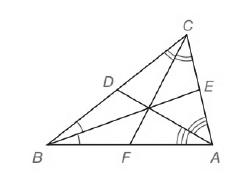Chapter 5.CT, Problem 14CT### Elementary Geometry for College St...

6th Edition
Daniel C. Alexander + 1 other
ISBN: 9781285195698

#### Solutions

Chapter
Section### Elementary Geometry for College St...

6th Edition
Daniel C. Alexander + 1 other
ISBN: 9781285195698
Textbook Problem
1 views

# For △ A B C , the three angle bisectors are shown.Find the product A E E C · C D D B · B F F A . _________________To determine

To find:

The product of AEEC·CDDB·BFFA

Explanation

Given, ABC,

The three angle bisectors are shown below,

Ceva’s Theorem:

Let point D be any point in the interior of ABC, where E, F and G lie on ABC, let BE¯,AF¯ and CG¯ be the line segments determined by D and vertices of ABC

### Still sussing out bartleby?

Check out a sample textbook solution.

See a sample solution

#### The Solution to Your Study Problems

Bartleby provides explanations to thousands of textbook problems written by our experts, many with advanced degrees!

Get Started

#### State the Midpoint Rule.

Single Variable Calculus: Early Transcendentals

#### (x327y6)2/3

Applied Calculus for the Managerial, Life, and Social Sciences: A Brief Approach

#### Expand each expression in Exercises 122. (x+1x)2

Finite Mathematics and Applied Calculus (MindTap Course List)

#### In Exercises 9 and 10, find the limit. limx3(2x23x+4)

Calculus: An Applied Approach (MindTap Course List)

#### Solve each equation. x+3x-1-6x=1

College Algebra (MindTap Course List)

#### Define a construct and explain the role that constructs play in theories.

Research Methods for the Behavioral Sciences (MindTap Course List)